Next: Transistor Circuits Up: Diode Circuits Previous: Diode Protection

# Problems

1. Make a sketch showing the current through an ideal diode as a function of the applied voltage. Also sketch the current through a real signal diode as a function of voltage.
2. Make a sketch showing the current drawn through a Zener diode as a function of the applied voltage. Show how to determine the forward resistance (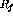), the reverse resistance (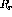) and the Zener resistance (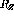) from your sketch. Label the voltages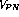and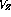.
1. Sketch the expected output waveforms when
1. a 100 Hz sine wave with a peak voltage of 5 V, and
2. a 100 Hz square wave with a peak-to-peak voltage of 10 V
are applied separately to each of the circuits below.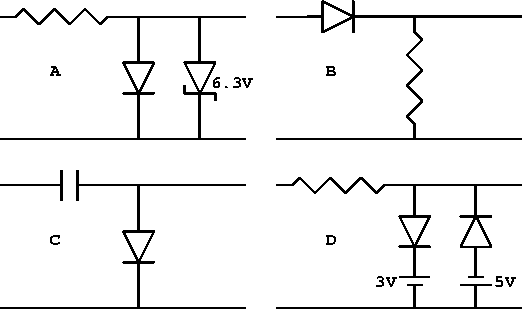2. The Zener diode in the following circuit is characterized by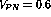V and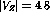V. Terminal B is at ground and there is no external load resistor.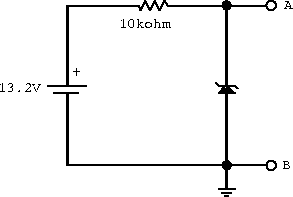1. What is the voltage at terminal A in the above circuit?
2. What is the current through the Zener? (What reasonable approximation makes this straightforward?).
3. If the Zener is dissipating 1.0W (in heat), what can we conclude to be the effective impedance of the diode for this situation?
3. The effects of a Zener diode in a circuit can be treated analytically by using an equivalent circuit model of the reverse-biased condition. In the low voltage region, before breakdown, the Zener diode can be treated like any other reverse-biased diode. However, the Zener diode is normally operated in the breakdown region.
1. Write down an equivalent linear-circuit model in the breakdown voltage region.
2. Replace the Zener diode in the following voltage reference circuit by your equivalent circuit model.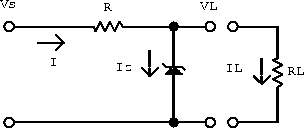3. Determine the contribution of the of Zener diode to this voltage reference circuit by calculating the elements of a Thevenin equivalent circuit representation.
4. Show that for small Zener diode effective resistance the Thevenin equivalent voltage is close to the Zener breakdown voltage and thus is insensitive to changes in the source voltage.
5. Show that for small Zener diode effective resistance, the Thevenin equivalent resistor gives the voltage source a reasonably small output impedance.
6. The combined results show a voltage reference that is insensitive to voltage changes in the original EMF and to changes in the load current. State the assumptions under which this result is valid.Next: Transistor Circuits Up: Diode Circuits Previous: Diode Protection

Doug Gingrich
Tue Jul 13 16:55:15 EDT 1999